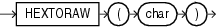## HEXTORAW

SyntaxDescription of the illustration hextoraw.eps

Purpose

`HEXTORAW` converts `char` containing hexadecimal digits in the `CHAR`, `VARCHAR2`, `NCHAR`, or `NVARCHAR2` data type to a raw value.

This function does not support `CLOB` data directly. However, `CLOB`s can be passed in as arguments through implicit data conversion.

Examples

The following example creates a simple table with a raw column, and inserts a hexadecimal value that has been converted to `RAW`:

```CREATE TABLE test (raw_col RAW(10));

INSERT INTO test VALUES (HEXTORAW('7D'));
```

The following example converts hexadecimal digits to a raw value and casts the raw value to `VARCHAR2`:

```SELECT UTL_RAW.CAST_TO_VARCHAR2(HEXTORAW('4041424344'))
FROM DUAL;

UTL_RAW.CAST_TO_VARCHAR2(HEXTORAW('4041424344'))
------------------------------------------------
@ABCD```